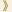HomeShaw Laureates2005Mathematical SciencesPress Release

The equation

x2 + y2 = z2

has infinitely many solutions for which x, y and z are positive integers. The smallest such solution is

32 + 42 = 52

which has been known since antiquity. In 1630 Fermat (1601-1665) conjectured that the more general equation

xn + yn = zn, for n = integer > 2,

has no integer solutions. This was later called Fermat's last theorem. It remained the most famous unproven conjecture in mathematics for more than three centuries until 1994 when Wiles completed his long and difficult proof, which uses powerful mathematical ideas and insights developed in the 19th and 20th centuries.

Mathematical Sciences Selection Committee
The Shaw Prize

2 September 2005, Hong Kong